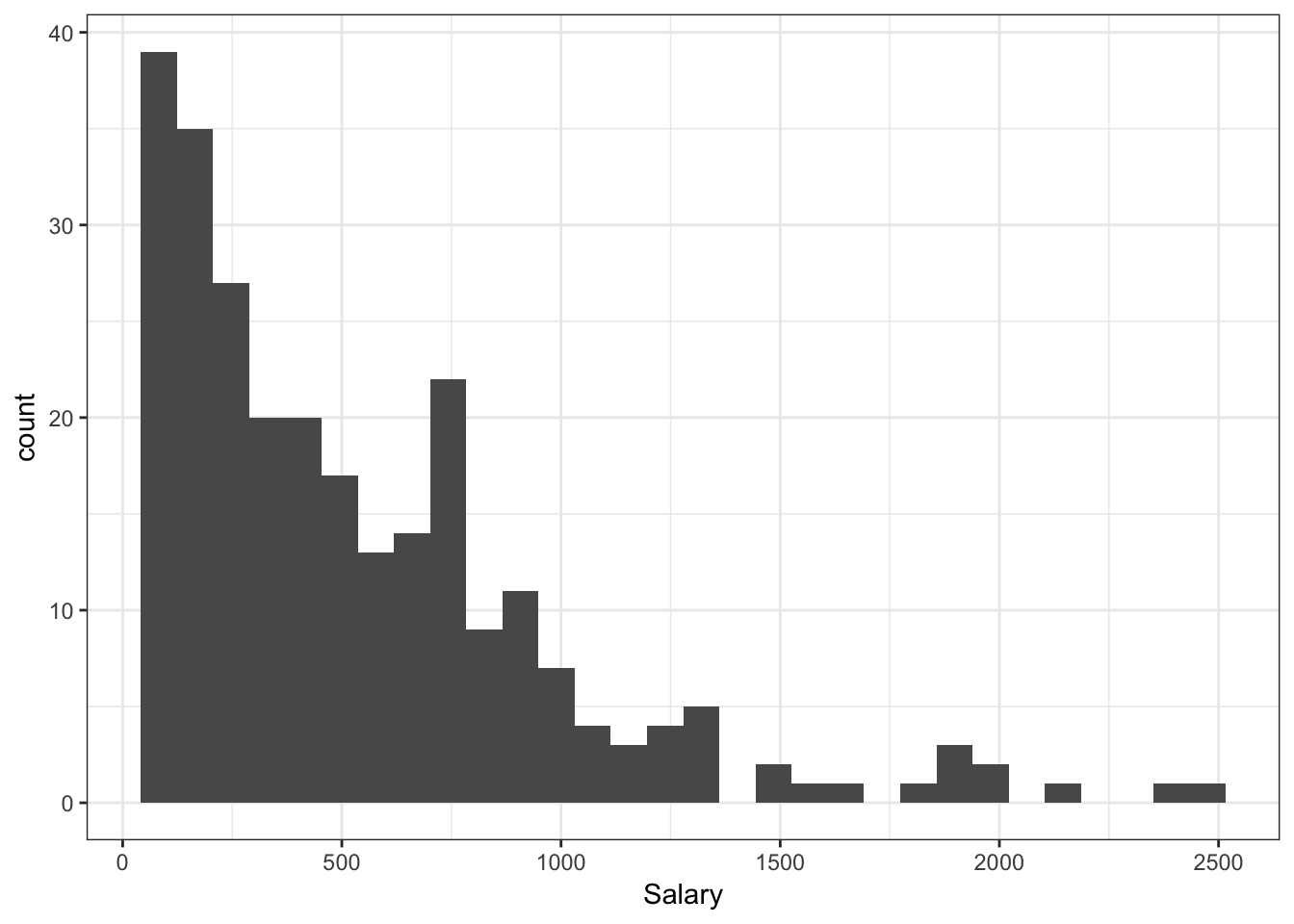``````library(ggplot2) # graphics library
library(ISLR)    # contains code and data from the textbook``````
``## Warning: package 'ISLR' was built under R version 3.4.2``
``````library(knitr)   # contains kable() function
library(leaps)   # for regsubsets() function
library(boot)    # for cv.glm
library(gam)``````
``## Warning: package 'gam' was built under R version 3.4.4``
``## Loading required package: splines``
``## Loading required package: foreach``
``## Loaded gam 1.16``
``library(glmnet)``
``## Loading required package: Matrix``
``## Loaded glmnet 2.0-10``
``options(scipen = 4)  # Suppresses scientific notation``

### 2. Best Subset Selection

This portion of the lab gets you to carry out the Lab in §6.5.1 of ISLR (Pages 244 - 247). You will want to have the textbook Lab open in front you as you go through these exercises. The ISLR Lab provides much more context and explanation for what you’re doing.

You will need the `Hitters` data set from the `ISLR` library in order to complete this exercise.

Please run all of the code indicated in §6.5.1 of ISLR, even if I don’t explicitly ask you to do so in this document.

##### Run the `View()` command on the `Hitters` data to see what the data set looks like.
``#View(Hitters)``
##### (a) Use `qplot` to construct a histogram of of the `Salary` variable. Does `Salary` appear to be normally distributed, or is the distribution skewed? What units is `Salary` recorded in?
``qplot(data = Hitters, x = Salary) + theme_bw()``
``## `stat_bin()` using `bins = 30`. Pick better value with `binwidth`.``
``## Warning: Removed 59 rows containing non-finite values (stat_bin).``• `Salary` denotes a player’s 1987 annual salary as recorded on the opening day of the season. This variable is measured in thousands of dollars. i.e., `Salary = 1500` corrsponds to a salary of \$1.5million.
##### (b) Below is a modified `panel.cor` function that properly handles missing values. Use the `pairs` command to construct a pairs plot for the Hitters data, displaying correlations in the lower panel and plots in the upper panel. Your pairs plot should include the variables: `Salary`, `AtBat`, `Hits`, `HmRun`, `CRBI`, `RBI`, `Errors`. Read the `?Hitters` documentation to understand what these variables mean.
``````panel.cor <- function(x, y, digits = 2, prefix = "", cex.cor, ...) {
usr <- par("usr"); on.exit(par(usr))
par(usr = c(0, 1, 0, 1))
r <- abs(cor(x, y, use = "complete.obs"))
txt <- format(c(r, 0.123456789), digits = digits)
txt <- paste0(prefix, txt)
if(missing(cex.cor)) cex.cor <- 0.8/strwidth(txt)
text(0.5, 0.5, txt, cex = cex.cor * r)
}

pairs(Hitters[, c("Salary", "AtBat", "Hits", "HmRun", "CRBI", "RBI", "Errors")],
lower.panel = panel.cor)``````